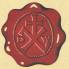Wacker Art Abstract AlgebraBild: "Infinit Sets"

Definition of a Group

A group G is a set equipped with an associative operation on two elements a, b of G that will result in a third element c of G. A group has an invers for each element and a neutral element for the operation.

Definition

• A group is a pair (G, "○" ) consisting of a set G and an operation "○".

• The operation a○b -> c maps each pair of elements (a,b), with a, b ∈ G to a third element c ∈ G.

• The set G shall be equipped with a neutral element e G with the property: e○a = a○e = a.

• For each element their shall be an invers element a-1G with the properties a○a-1 = a-1○a = e.

• Associative Property of the operation "○": (a○b)○c = a○(b○c).

Example

The set ℤ of the integer numbers and the operation "+" form a group (ℤ "+" ).

The operation a+b -> c; for all a,b ∈ ℤ will have the result c ∈ ℤ.

a+0 = 0+a = a; for all a ∈ ℤ; with 0 as the neutral element of ℤ for the operation "+".

a+(-a) = (-a)+a = 0; The element (-a) is the invers of a with (-a), a ∈ ℤ.

(a+b)+c = a+(b+c); with a, b, c ∈ ℤ.

Commutative Group - Abelian Group

An Abelian group is a group, where the group operation "○" is commutative.

a ○ b = b ○ a for all a,b ∈ G;

If the group operation is commutative for all elements of the group set, the group is called Abelian.

Non Commutative Group - Non-Abelian Group

The definition of a group does not require, that the operation "○" is commutative.

It is possible that a ○ b ≠ b ○ a for some elements a, b ∈ G;

A group where the group operation is not commutative is called a non-Abelian group or non commutative group.

Lie Group

A Lie Group is as a Manifold M together with a product φ(x,y).

Definition

• A Lie Group is a pair (M, "φ") consisting of a manifold M and a product "φ";

Closer: φ(x,y) ∈ M for all x,y ∈ M;

Neutral Element: It exists an element e ∈ M called the neutral element of the operation φ; with φ(e,x) = φ(x,e) = x;

Invers: For every element x ∈ M there exists a unique element xM such that φ(x,x) = φ(x,x) = e;

Associativity: φ(φ(x,y),z) = φ(x,φ(y,z) for all x,y,z ∈ M.

Many of the group properties can be uncovered by examing the group properties near the identity element of a Lie group. The product of a Lie group near the identity element induces a Lie-Algebra with a commutator braket structure at the tangent space.

Axiomatic Definition of a Vector Space

Axioms of a vector space.

Definition:

A vector space 𝕍 is a set of objects called vectors.

There are two operations defined on 𝕍:
Scalar multiplication av and vector addition: v+w.

There is a special member 0 ∈ 𝕍.

The following Axioms are satisfied for all vectors u,v,w ∈ 𝕍 and all scalars a,b ∈ ℝ

 V1. av ∈ 𝕍, v+w ∈ 𝕍 𝕍 is closed under scalar multiplication and vector addition. V2. v+w = w+v Vector addition is commutative. V3. (u+v)+w = u+(v+w) Vector addition is associative. V4. v+0 = v 0 is the additive identity V5. 0v = 0 V6. 1v = v 1 is the multiplicative identity. V7. a(bv) = (ab)v V8. a(v+w) = av + aw Scalar multiplication is distributiv over vector addition. V9. (a+b)v = av + bv

Next Page:

5. März 2021 Version 2.0
Copyright: Hermann Wacker Uhlandstraße 10 D-85386 Eching bei Freising Germany Haftungsausschluß